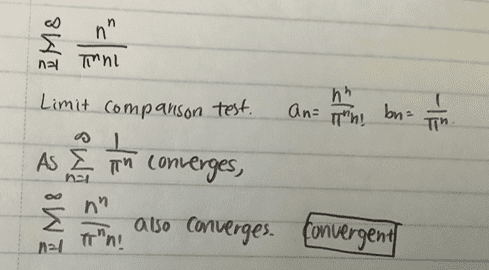# Determining if series converges or diverges

• Sunwoo Bae

#### Sunwoo Bae

Homework Statement
Determine if the following series converges or diverges using any appropriate tests
Relevant Equations
limit comparison testIs it valid to use limit comparison test to compute the following series?
If it is, would my reasoning be valid?

Thank you!

•Delta2

An orthodox way is to investigate
$$|\frac{a_{n+1}}{a_n}|$$
is greater or less than 1.

•Delta2
Homework Statement:: Determine if the following series converges or diverges using any appropriate tests
Relevant Equations:: limit comparison test

View attachment 290042
Is it valid to use limit comparison test to compute the following series?
If it is, would my reasoning be valid?

Thank you!
What does the limit comparison test say exactly?
Isn't there a specific relation to be fulfilled between ##a_n## and ##b_n##?
Do your successions ##a## and ##b## fulfill such relation?

•Delta2
Homework Statement:: Determine if the following series converges or diverges using any appropriate tests
Relevant Equations:: limit comparison test

View attachment 290042
Is it valid to use limit comparison test to compute the following series?
If it is, would my reasoning be valid?

Thank you!

Is $$\frac{n^n}{n!} = \frac{n}{1} \frac{n}{2} \cdots \frac{n}{n-1} \frac{n}{n}$$ greater than, or less than, 1 for large $n$? Given that, which of the following is true:
1. $a_n < b_n$ for large $n$.
2. $a_n > b_n$ for large $n$.

How does that affect the comparison test?

•jim mcnamara and Delta2
I personally think the ratio test would be the easiest to use, here.